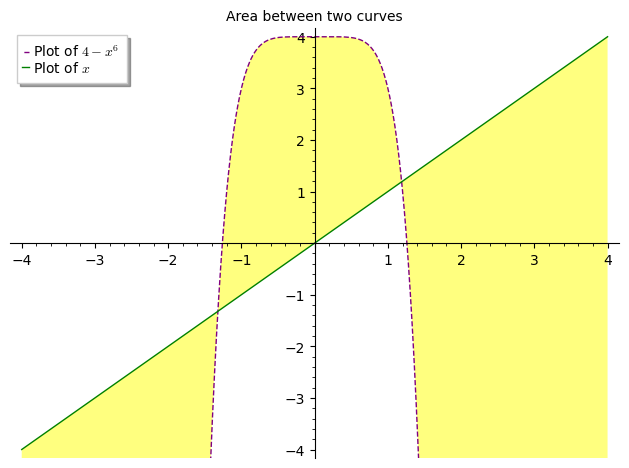# Area under two curves

I am trying to fill in the area between two functions, $F(x) = 4-x^6$ and $G(x) = x$.

This is my code so far. It won't fill properly, it takes into consideration other areas of the functions.

f(x) = 4 - x^6
g(x) = x

P1 = plot(f, (-4, 4), color='purple', linestyle="dashed", fill=True,
legend_label='Plot of $4-x^6$')
P2 = plot(g, (-4, 4), color='green', title="Area between two curves",
legend_label='Plot of $x$')

show(P1 + P2, ymin=-4, ymax=4)

edit retag close merge delete

Sort by » oldest newest most voted

Hello, @Sagematherireland! You are in the right track (you almost got it done!) The fill argument for the plot command can take on the value of a function, in which case, it will fill the area between the curves. Here is an example that I believe should produce the result you expected:

f(x) = 4 - x^6
g(x) = x
P1 = plot(f, (-4,4), color='purple', linestyle='dashed', fill=g, fillcolor='yellow', legend_label='Plot of $4-x^6$')
P2 = plot(g, (-4,4), color='green', title="Area between two curves", legend_label='Plot of $x$')
show(P1+P2, ymin=-4, ymax=4)


Notice I have changed the fill=True argument to fill=g. I also used the fillcolor argument in the first plot just for fun. Here is the result:Hope this helps!

more